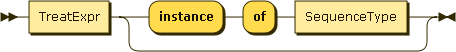### Instance-of expressions

Figure 63. InstanceofExprAn instance expression can be used to tell whether a JSONiq value matches a given sequence type.

Example 139. Instance of expression

```1 instance of integer
```

Result (run with Zorba): true

Example 140. Instance of expression

```1 instance of string
```

Result (run with Zorba): false

Example 141. Instance of expression

```"foo" instance of string
```

Result (run with Zorba): true

Example 142. Instance of expression

```{ "foo" : "bar" } instance of object
```

Result (run with Zorba): true

Example 143. Instance of expression

```({ "foo" : "bar" }, { "bar" : "foo" }) instance of json-item+
```

Result (run with Zorba): true

Example 144. Instance of expression

```[ 1, 2, 3 ] instance of array?
```

Result (run with Zorba): true

Example 145. Instance of expression

```() instance of ()
```

Result (run with Zorba): true